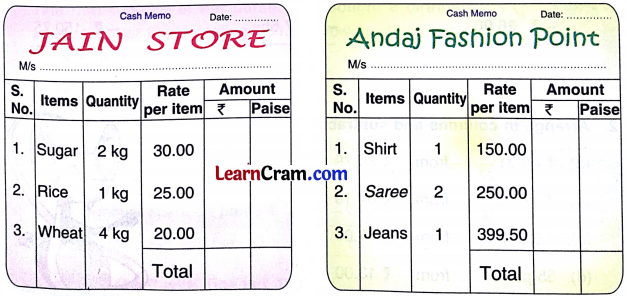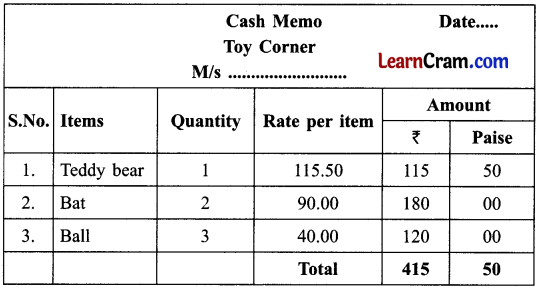# DAV Class 3 Maths Chapter 7 Worksheet 6 Solutions

The DAV Class 3 Maths Solutions and DAV Class 3 Maths Chapter 7 Worksheet 6 Solutions of Money offer comprehensive answers to textbook questions.

## DAV Class 3 Maths Ch 7 WS 6 Solutions

Question 1.
Look at the cash memo and answer the questions.(a) How many bats were purchased?
Solution:
2 bats

(b) What is the cost of 1 teddy bear?
Solution:
₹ 50.50

(c) What is the total amount?
Solution:
₹ 600.50Question 2.
Look at the cash memo and answer the questions.(a) What is the name of the shop?
Solution:
Aggarwal Sweet Comer.

(b) How many kilograms of burfi was purchased?
Solution:
2 kg Burfi was purchased.

(e) What is the cost of 1 kg Jalebi?
Solution:
₹ 65

(d) What is the total amount?
Solution:
₹ 855Question 3.
Compute the following cash memos. (See P-89 Textbook)Solution:### DAV Class 3 Maths Chapter 3 Worksheet 6 Notes

Add amounts of money on cash memo

Question 1.
Look at the cash memo and answer the questions.(a) How many Balls were purchased?
Solution:
3 balls

(b) What is the cost of two bats?
Solution:
₹ 180

(c) What is the total amount?
Solution:
₹ 415.50